# Discussion 7: Hypothesis Testing and Permutation Testing

The problems in this worksheet are taken from past exams. Work on them on paper, since the exams you take in this course will also be on paper.

We encourage you to complete this worksheet in a live discussion section. Solutions will be made available after all discussion sections have concluded. You don’t need to submit your answers anywhere.

Note: We do not plan to cover all problems here in the live discussion section; the problems we don’t cover can be used for extra practice.

## Problem 1

For this question, we will use data from the 2021 Women’s National Basketball Association (WNBA) season for the next several problems. In basketball, players score points by shooting the ball into a hoop. The team that scores the most points wins the game.

Kelsey Plum, a WNBA player, attended La Jolla Country Day School, which is adjacent to UCSD’s campus. Her current team is the Las Vegas Aces (three-letter code 'LVA'). In 2021, the Las Vegas Aces played 31 games, and Kelsey Plum played in all 31.

The DataFrame plum contains her stats for all games the Las Vegas Aces played in 2021. The first few rows of plum are shown below (though the full DataFrame has 31 rows, not 5):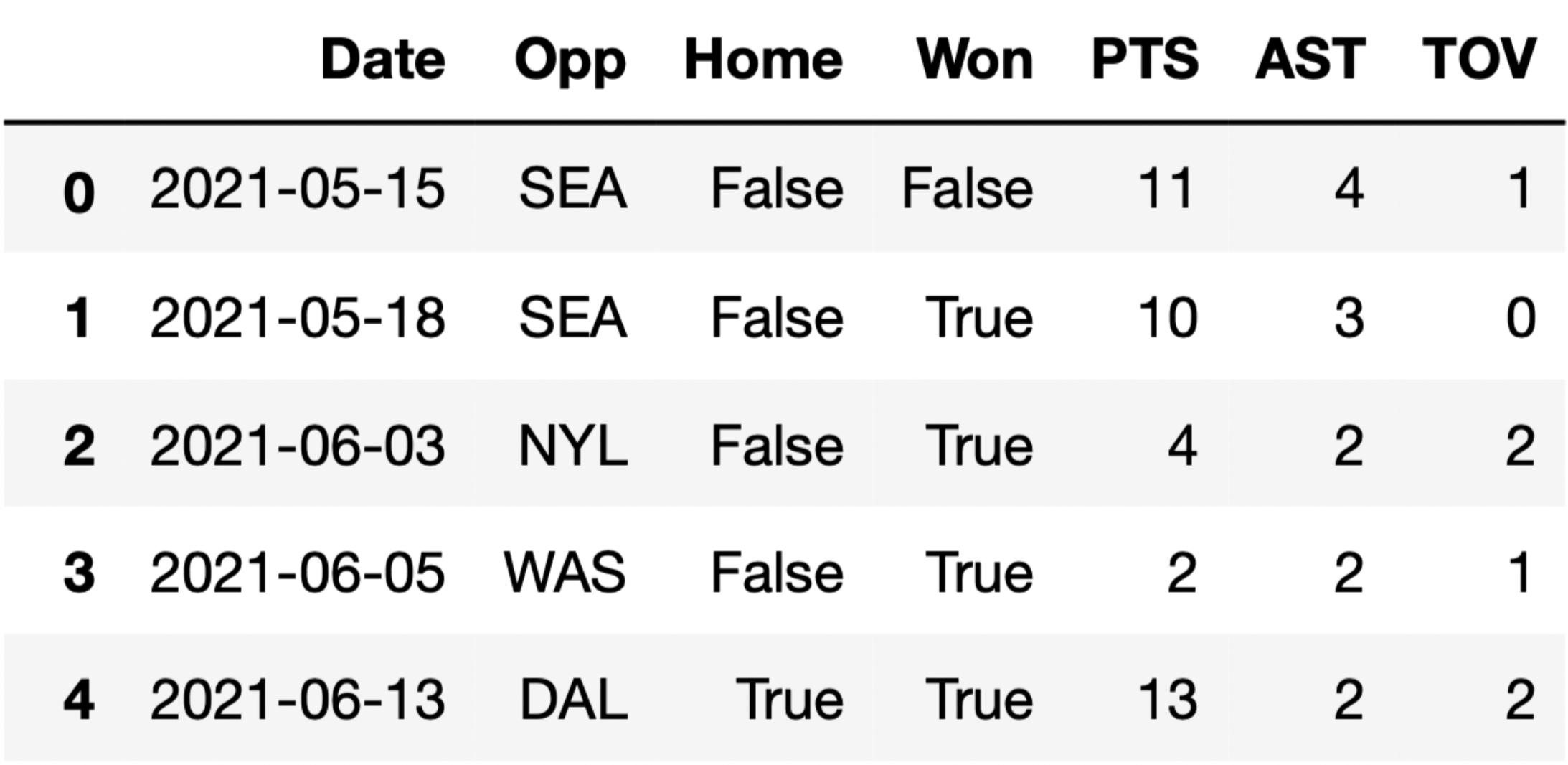Each row in plum corresponds to a single game. For each game, we have:

• 'Date' (str), the date on which the game was played
• 'Opp' (str), the three-letter code of the opponent team
• 'Home' (bool), True if the game was played in Las Vegas (“home”) and False if it was played at the opponent’s arena (“away”)
• 'Won' (bool), True if the Las Vegas Aces won the game and False if they lost
• 'PTS' (int), the number of points Kelsey Plum scored in the game
• 'AST' (int), the number of assists (passes) Kelsey Plum made in the game
• 'TOV' (int), the number of turnovers Kelsey Plum made in the game (a turnover is when you lose the ball – turnovers are bad!)

Consider the definition of the function diff_in_group_means:

def diff_in_group_means(df, group_col, num_col):
s = df.groupby(group_col).mean().get(num_col)
return s.loc[False] - s.loc[True]

### Problem 1.1

It turns out that Kelsey Plum averages 0.61 more assists in games that she wins than in games that she loses. After observing that Kelsey Plum averages more assists in winning games than in losing games, we become interested in conducting a permutation test for the following hypotheses:

• Null Hypothesis: The number of assists Kelsey Plum makes in winning games and in losing games come from the same distribution.
• Alternative Hypothesis: The number of assists Kelsey Plum makes in winning games is higher on average than the number of assists that she makes in losing games.

To conduct our permutation test, we place the following code in a for-loop.


won = plum.get('Won')
ast = plum.get('AST')
shuffled = plum.assign(Won_shuffled=np.random.permutation(won)) \
.assign(AST_shuffled=np.random.permutation(ast))

Which of the following options does not compute a valid simulated test statistic for this permutation test?

• diff_in_group_means(shuffled, 'Won', 'AST')

• diff_in_group_means(shuffled, 'Won', 'AST_shuffled')

• diff_in_group_means(shuffled, 'Won_shuffled, 'AST')

• diff_in_group_means(shuffled, 'Won_shuffled, 'AST_shuffled')

• More than one of these options do not compute a valid simulated test statistic for this permutation test

### Problem 1.2

Suppose we generate 10,000 simulated test statistics, using one of the valid options from Question 1.1. The empirical distribution of test statistics, with a red line at observed_diff, is shown below.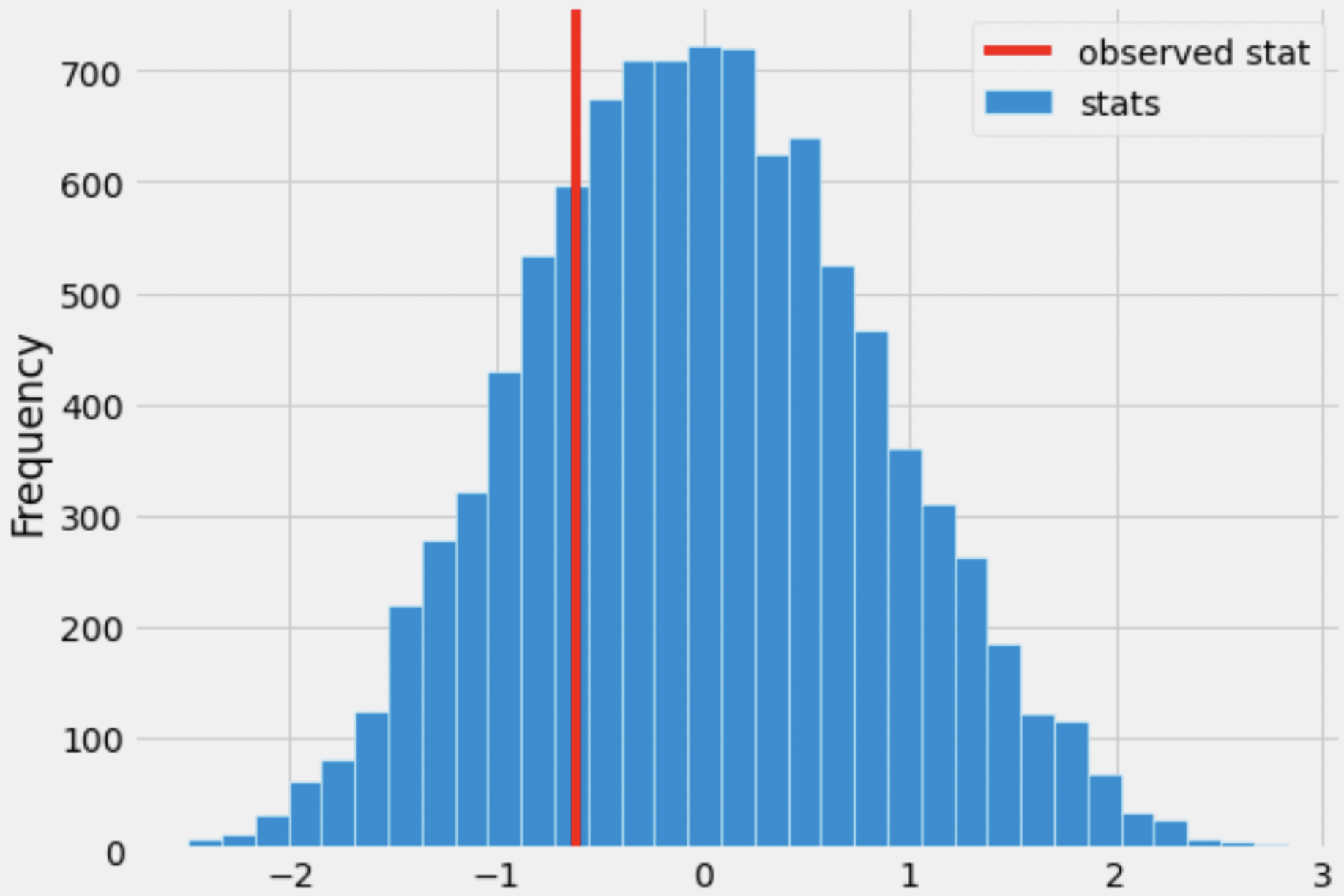Roughly one-quarter of the area of the histogram above is to the left of the red line. What is the correct interpretation of this result?

• There is roughly a one quarter probability that Kelsey Plum’s number of assists in winning games and in losing games come from the same distribution.

• The significance level of this hypothesis test is roughly a quarter.

• Under the assumption that Kelsey Plum’s number of assists in winning games and in losing games come from the same distribution, and that she wins 22 of the 31 games she plays, the chance of her averaging at least 0.61 more assists in wins than losses is roughly a quarter.

• Under the assumption that Kelsey Plum’s number of assists in winning games and in losing games come from the same distribution, and that she wins 22 of the 31 games she plays, the chance of her averaging 0.61 more assists in wins than losses is roughly a quarter.

## Problem 2

Let’s suppose there are 4 different types of shots a basketball player can take – layups, midrange shots, threes, and free throws.

The DataFrame breakdown has 4 rows and 50 columns – one row for each of the 4 shot types mentioned above, and one column for each of 50 different players. Each column of breakdown describes the distribution of shot types for a single player.

The first few columns of breakdown are shown below.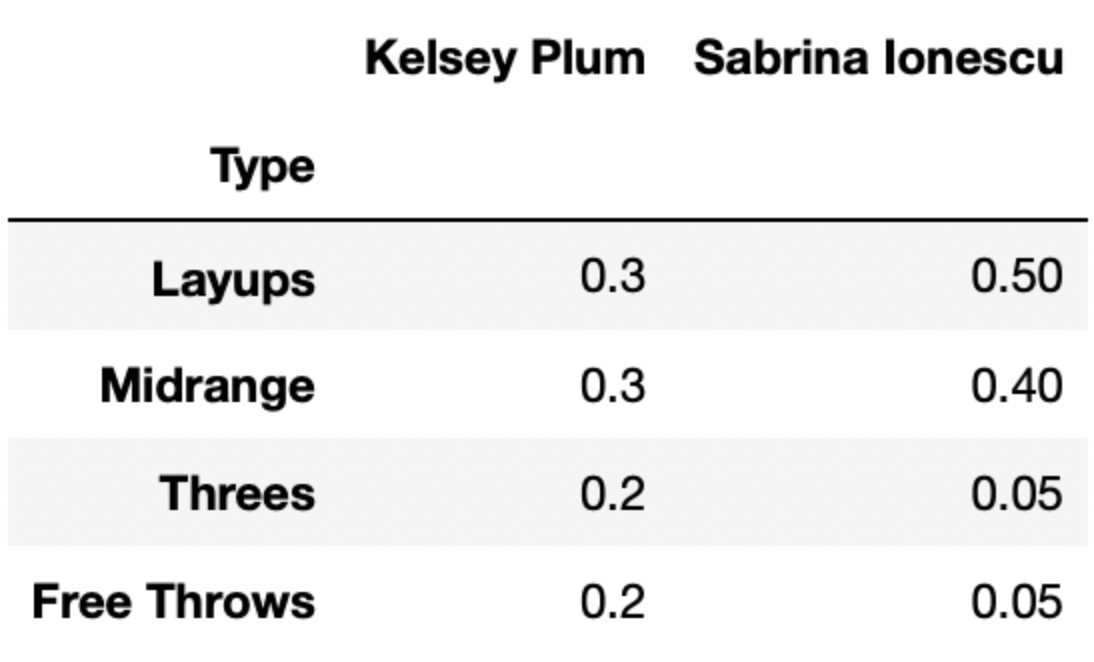For instance, 30% of Kelsey Plum’s shots are layups, 30% of her shots are midrange shots, 20% of her shots are threes, and 20% of her shots are free throws.

### Problem 2.1

Below, we’ve drawn an overlaid bar chart showing the shot distributions of Kelsey Plum and Chiney Ogwumike, a player on the Los Angeles Sparks.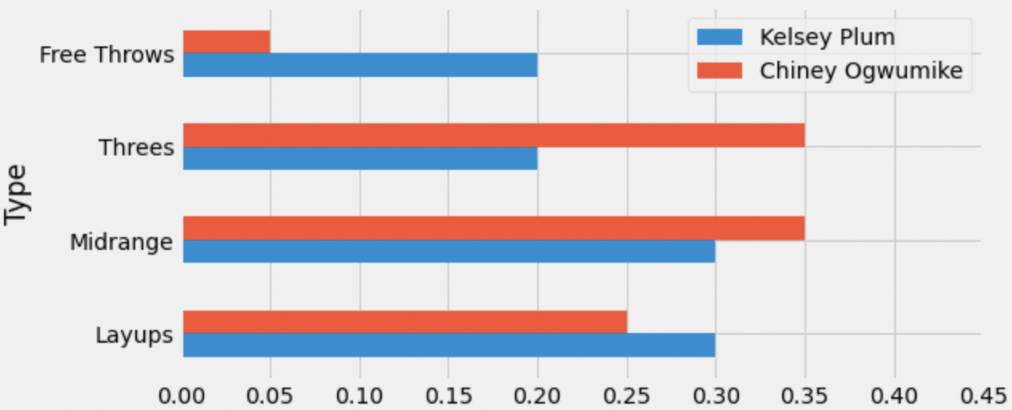What is the total variation distance (TVD) between Kelsey Plum’s shot distribution and Chiney Ogwumike’s shot distribution? Give your answer as a proportion between 0 and 1 (not a percentage) rounded to three decimal places.

### Problem 2.2

Recall, breakdown has information for 50 different players. We want to find the player whose shot distribution is the most similar to Kelsey Plum, i.e. has the lowest TVD with Kelsey Plum’s shot distribution.

Fill in the blanks below so that most_sim_player evaluates to the name of the player with the most similar shot distribution to Kelsey Plum. Assume that the column named 'Kelsey Plum' is the first column in breakdown (and again that breakdown has 50 columns total).

most_sim_player = ''
lowest_tvd_so_far = __(a)__
other_players = np.array(breakdown.columns).take(__(b)__)
for player in other_players:
player_tvd = tvd(breakdown.get('Kelsey Plum'),
breakdown.get(player))
if player_tvd < lowest_tvd_so_far:
lowest_tvd_so_far = player_tvd
__(c)__
1. What goes in blank (a)?
• -1

• -0.5

• 0

• 0.5

• 1

• np.array([])

• ''

1. What goes in blank (b)?

2. What goes in blank (c)?

### Problem 2.3

Let’s again consider the shot distributions of Kelsey Plum and Cheney Ogwumike.We define the maximum squared distance (MSD) between two categorical distributions as the largest squared difference between the proportions of any category.

What is the MSD between Kelsey Plum’s shot distribution and Chiney Ogwumike’s shot distribution? Give your answer as a proportion between 0 and 1 (not a percentage) rounded to three decimal places.

### Problem 2.4

For your convenience, we show the first few columns of breakdown again below.• layups are worth 2 points,
• midrange shots are worth 2 points,
• threes are worth 3 points, and
• free throws are worth 1 point

Suppose that Kelsey Plum is guaranteed to shoot exactly 10 shots a game. The type of each shot is drawn from the 'Kelsey Plum' column of breakdown (meaning that, for example, there is a 30% chance each shot is a layup).

Fill in the blanks below to complete the definition of the function simulate_points, which simulates the number of points Kelsey Plum scores in a single game. (simulate_points should return a single number.)

def simulate_points():
shots = np.random.multinomial(__(a)__, breakdown.get('Kelsey Plum'))
possible_points = np.array([2, 2, 3, 1])
return __(b)__
1. What goes in blank (a)?
2. What goes in blank (b)?

## Problem 3

IKEA is a Swedish furniture company that designs and sells ready-to-assemble furniture and other home furnishings.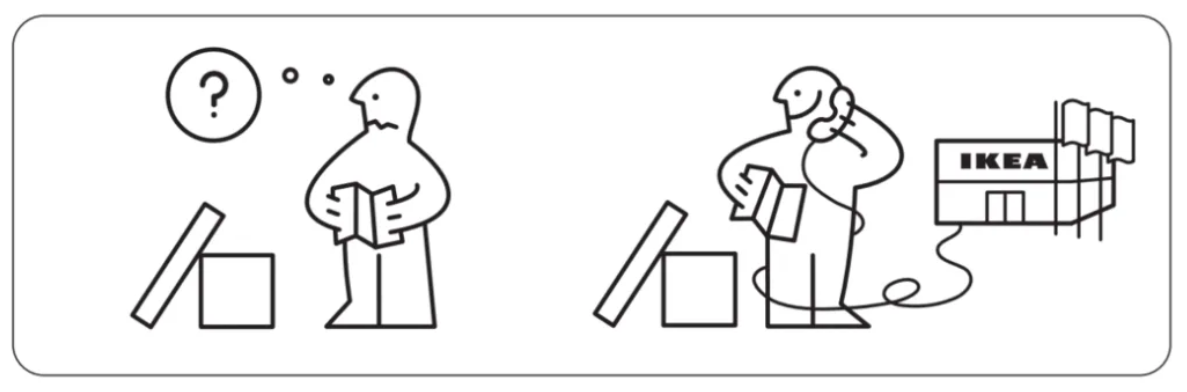An IKEA fan created an app where people can log the amount of time it took them to assemble their IKEA furniture. The DataFrame app_data has a row for each product build that was logged on the app. The columns are:

• 'product' (str): the name of the product, which includes the product line as the first word, followed by a description of the product
• 'category' (str): a categorical description of the type of product
• 'assembly_time' (str): the amount of time to assemble the product, formatted as 'x hr, y min' where x and y represent integers, possibly zero

The first few rows of app_data are shown below, though app_data has many more rows than pictured (5000 rows total).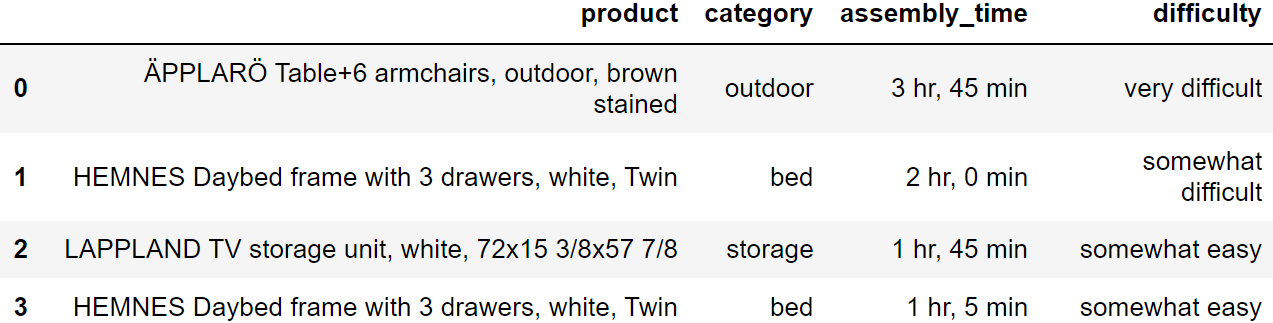Assume that we have already run import babypandas as bpd and import numpy as np.

You are browsing the IKEA showroom, deciding whether to purchase the BILLY bookcase or the LOMMARP bookcase. You are concerned about the amount of time it will take to assemble your new bookcase, so you look up the assembly times reported in app_data. Thinking of the data in app_data as a random sample of all IKEA purchases, you want to perform a permutation test to test the following hypotheses.

Null Hypothesis: The assembly time for the BILLY bookcase and the assembly time for the LOMMARP bookcase come from the same distribution.

Alternative Hypothesis: The assembly time for the BILLY bookcase and the assembly time for the LOMMARP bookcase come from different distributions.

### Problem 3.1

Suppose we added a column to app_data called 'minutes', containing the 'assembly_time' value for each entry converted to an integer amount of minutes. Then, we query app_data to keep only the BILLY bookcases, then average the 'minutes' column. In addition, we separately query app_data to keep only the LOMMARP bookcases, then average the 'minutes' column. If the null hypothesis is true, which of the following statements about these two averages is correct?

• These two averages are the same.

• Any difference between these two averages is due to random chance.

• Any difference between these two averages cannot be ascribed to random chance alone.

• The difference between these averages is statistically significant.

### Problem 3.2

For the permutation test, we’ll use as our test statistic the average assembly time for BILLY bookcases minus the average assembly time for LOMMARP bookcases, in minutes.

Complete the code below to generate one simulated value of the test statistic in a new way, without using np.random.permutation.

billy = (app_data.get('product') ==
'BILLY Bookcase, white, 31 1/2x11x79 1/2')
lommarp = (app_data.get('product') ==
'LOMMARP Bookcase, dark blue-green, 25 5/8x78 3/8')
billy_lommarp = app_data[billy|lommarp]
billy_mean = np.random.choice(billy_lommarp.get('minutes'), billy.sum()).mean()
lommarp_mean = _________
billy_mean - lommarp_mean

What goes in the blank?

• billy_lommarp[lommarp].get('minutes').mean()

• np.random.choice(billy_lommarp.get('minutes'), lommarp.sum()).mean()

• billy_lommarp.get('minutes').mean() - billy_mean

• (billy_lommarp.get('minutes').sum() - billy_mean * billy.sum())/lommarp.sum()

## Problem 4

Researchers from the San Diego Zoo, located within Balboa Park, collected physical measurements of three species of penguins (Adelie, Chinstrap, or Gentoo) in a region of Antarctica. One piece of information they tracked for each of 330 penguins was its mass in grams. The average penguin mass is 4200 grams, and the standard deviation is 840 grams.

We’re interested in investigating the differences between the masses of Adelie penguins and Chinstrap penguins. Specifically, our null hypothesis is that their masses are drawn from the same population distribution, and any observed differences are due to chance only.

Below, we have a snippet of working code for this hypothesis test, for a specific test statistic. Assume that adelie_chinstrap is a DataFrame of only Adelie and Chinstrap penguins, with just two columns – 'species' and 'mass'.

stats = np.array([])
num_reps = 500
for i in np.arange(num_reps):
# --- line (a) starts ---
# --- line (a) ends ---

# --- line (b) starts ---
# --- line (b) ends ---

grouped = with_shuffled.groupby('species').mean()

# --- line (c) starts ---
stat = grouped.get('mass').iloc - grouped.get('mass').iloc
# --- line (c) ends ---

stats = np.append(stats, stat)

### Problem 4.1

Which of the following statements best describe the procedure above?

• This is a standard hypothesis test, and our test statistic is the total variation distance between the distribution of Adelie masses and Chinstrap masses

• This is a standard hypothesis test, and our test statistic is the difference between the expected proportion of Adelie penguins and the proportion of Adelie penguins in our resample

• This is a permutation test, and our test statistic is the total variation distance between the distribution of Adelie masses and Chinstrap masses

• This is a permutation test, and our test statistic is the difference in the mean Adelie mass and mean Chinstrap mass

### Problem 4.2

Currently, line (c) (marked with a comment) uses .iloc. Which of the following options compute the exact same statistic as line (c) currently does?

Option 1:

stat = grouped.get('mass').loc['Adelie'] - grouped.get('mass').loc['Chinstrap']

Option 2:

stat = grouped.get('mass').loc['Chinstrap'] - grouped.get('mass').loc['Adelie']
• Option 1 only

• Option 2 only

• Both options

• Neither option

### Problem 4.3

Is it possible to re-write line (c) in a way that uses .iloc twice, without any other uses of .loc or .iloc?

• Yes, it’s possible

• No, it’s not possible

### Problem 4.4

For your convenience, we copy the code for the hypothesis test below.

stats = np.array([])
num_reps = 500
for i in np.arange(num_reps):
# --- line (a) starts ---
# --- line (a) ends ---

# --- line (b) starts ---
# --- line (b) ends ---

grouped = with_shuffled.groupby('species').mean()

# --- line (c) starts ---
stat = grouped.get('mass').iloc - grouped.get('mass').iloc
# --- line (c) ends ---

stats = np.append(stats, stat)

What would happen if we removed line (a), and replaced line (b) with

with_shuffled = adelie_chinstrap.sample(adelie_chinstrap.shape, replace=False)

• This would still run a valid hypothesis test

• This would not run a valid hypothesis test, as all values in the stats array would be exactly the same

• This would not run a valid hypothesis test, even though there would be several different values in the stats array

• This would not run a valid hypothesis test, as it would incorporate information about Gentoo penguins

### Problem 4.5

For your convenience, we copy the code for the hypothesis test below.

stats = np.array([])
num_reps = 500
for i in np.arange(num_reps):
# --- line (a) starts ---
# --- line (a) ends ---

# --- line (b) starts ---
# --- line (b) ends ---

grouped = with_shuffled.groupby('species').mean()

# --- line (c) starts ---
stat = grouped.get('mass').iloc - grouped.get('mass').iloc
# --- line (c) ends ---

stats = np.append(stats, stat)

What would happen if we removed line (a), and replaced line (b) with

with_shuffled = adelie_chinstrap.sample(adelie_chinstrap.shape, replace=True)

• This would still run a valid hypothesis test

• This would not run a valid hypothesis test, as all values in the stats array would be exactly the same

• This would not run a valid hypothesis test, even though there would be several different values in the stats array

• This would not run a valid hypothesis test, as it would incorporate information about Gentoo penguins

### Problem 4.6

For your convenience, we copy the code for the hypothesis test below.

stats = np.array([])
num_reps = 500
for i in np.arange(num_reps):
# --- line (a) starts ---
# --- line (a) ends ---

# --- line (b) starts ---
# --- line (b) ends ---

grouped = with_shuffled.groupby('species').mean()

# --- line (c) starts ---
stat = grouped.get('mass').iloc - grouped.get('mass').iloc
# --- line (c) ends ---

stats = np.append(stats, stat)

What would happen if we replaced line (a) with

with_shuffled = adelie_chinstrap.assign(
)

and replaced line (b) with

with_shuffled = with_shuffled.assign(
)

• This would still run a valid hypothesis test

• This would not run a valid hypothesis test, as all values in the stats array would be exactly the same

• This would not run a valid hypothesis test, even though there would be several different values in the stats array

• This would not run a valid hypothesis test, as it would incorporate information about Gentoo penguins

### Problem 4.7

Suppose we run the code for the hypothesis test and see the following empirical distribution for the test statistic. In red is the observed statistic.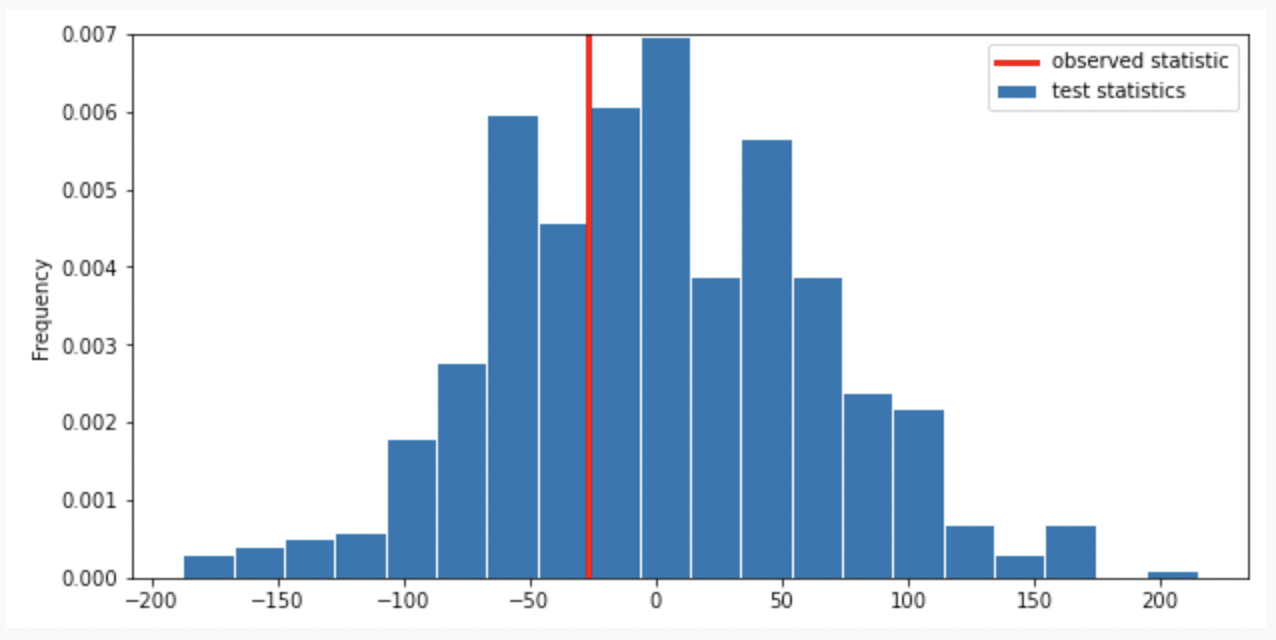Suppose our alternative hypothesis is that Chinstrap penguins weigh more on average than Adelie penguins. Which of the following is closest to the p-value for our hypothesis test?

• 0

• \frac{1}{4}

• \frac{1}{3}

• \frac{2}{3}

• \frac{3}{4}

• 1